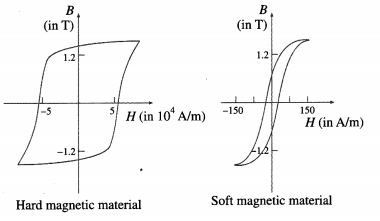# Maharashtra Board Class 12 Physics Solutions Chapter 11 Magnetic Materials

Balbharti Maharashtra State Board 12th Physics Textbook Solutions Chapter 11 Magnetic Materials Textbook Exercise Questions and Answers.

## Maharashtra State Board 12th Physics Solutions Chapter 11 Magnetic Materials

1. Choose the correct option.

i) Intensity of magnetic field of the earth at the point inside a hollow iron box is.
(A) less than that outside
(B) more than that outside
(C) same as that outside
(D) zero
(D) zero

ii) Soft iron is used to make the core of the transformer because of its
(A) low coercivity and low retentivity
(B) low coercivity and high retentivity
(C) high coercivity and high retentivity
(D) high coercivity and low retentivity
(A) low coercivity and low retentivityiii) Which of the following statements is correct for diamagnetic materials?
(A) µr < 1
(B) χ is negative and low
(C) χ does not depend on temperature
(D) All of above
(D) All of above

iv) A rectangular magnet suspended freely has a period of oscillation equal to T. Now it is broken into two equal halves ( each having half of the original length) and one piece is made to oscillate freely. Its period of oscillation is T′, the ratio of T′ / T is.
(A) $$\frac{1}2 \sqrt{2}$$
(B) $$\frac{1}{2}$$
(C) 2
(D) $$\frac{1}{4}$$
(B) $$\frac{1}{2}$$

v) A magnetising field of 360 Am -1 produces a magnetic flux density (B ) = 0.6 T in a ferromagnetic material. What is its permeability in Tm A-1 ?
(A) $$\frac{1}{300}$$
(B) 300
(C) $$\frac{1}{600}$$
(D) 600
(C) $$\frac{1}{600}$$

i) Which property of soft iron makes it useful for preparing electromagnet?
An electromagnet should become magnetic when a current is passed through its coil but should lose its magnetism once the current is switched off. Hence, the ferromagnetic core (usually iron-based) used for an electromagnet should have high permeability and low retentivity, i.e., it should be magnetically ‘soft’.

ii) What happens to a ferromagnetic material when its temperature increases above curie temperature?
A ferromagnetic material is composed of small regions called domains. Within each domain, the atomic magnetic moments of nearest-neighbour atoms interact strongly through exchange interaction, a quantum mechanical phenomenon, and align themselves parallel to each other even in the absence of an external magnetic field. A domain is, therefore, spontaneously magnetized to saturation.

The material retains its domain structure only up to a certain temperature. On heating, the increased thermal agitation works against the spontaneous domain magnetization. Finally, at a certain critical temperature, called the Curie point or Curie temperature, thermal agitation overcomes the exchange forces and keeps the atomic magnetic moments randomly oriented. Thus, above the Curie point, the material becomes paramagnetic. The ferromagnetic to paramagnetic transition is an order to disorder transition. When cooled below the Curie point, the material becomes ferromagnetic again.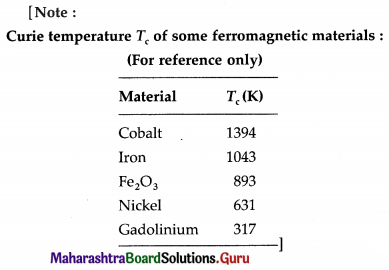iii) What should be retentivity and coercivity of permanent magnet?
A permanent magnet should have a large zero-field magnetization and should need a very large reverse field to demagnetize. In other words, it should have a very broad hysteresis loop with high retentivity and very high coercivity.iv) Discuss the Curie law for paramagnetic material.
Curie’s law : The magnetization of a paramagnetic material is directly proportional to the external magnetic field and inversely proportional to the absolute temperature of the material.

If a paramagnetic material at an absolute temperature T is placed in an external magnetic field of induction , the magnitude of its magnetization
Mz ∝ $$\frac{B_{\text {ext }}}{T}$$ ∴ Mz = C$$\frac{B_{\text {ext }}}{T}$$
where the proportionality constant C is called the Curie constant.
[Notes : (1) The above law, discovered experimentally in 1895 by Pierre Curie (1859-1906) French physcist, is true only for values of Bext/ T below about 0.5 tesla per kelvin.
(2) [C] = [Mz ∙ T] / [Bext] = [L-1I ∙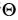] /[MT-2I-1]
= [M-1L-1T2I2],
wheredenotes the dimension of temperature.]

v) Obtain and expression for orbital magnetic moment of an electron rotating about the nucleus in an atom.
In the Bohr model of a hydrogen atom, the electron of charge – e performs a uniform circular motion around the positively charged nucleus. Let r, v and T be the orbital radius, speed and period of motion of the electron. Then,
T = $$\frac{2 \pi r}{v}$$ …………….. (1)
Therefore, the orbital magnetic moment asso-ciated with this orbital current loop has a magnitude,
I = $$\frac{e}{T}=\frac{e v}{2 \pi r}$$ …………… (2)
Therefore, the magnetic dipole moment associated with this electronic current loop has a magnitude
M0 = current × area of the loop
= I(πr2) = $$\frac{e v}{2 \pi r}$$ × πr2 = $$\frac{1}{2}$$ evr ……………… (3)
Multiplying and dividing the right hand side of the above expression by the electron mass me,
M0 = $$\frac{e}{2 m_{\mathrm{e}}}$$ (mevr) = $$\frac{e}{2 m_{\mathrm{e}}}$$ L0 ……………. (4)
where L0 = mevr is the magnitude of the orbital angular momentum of the electron. $$\vec{M}_{0}$$ is opposite to $$\vec{L}_{0}$$.
∴ $$\vec{M}_{0}=-\frac{e}{2 m_{e}} \overrightarrow{L_{0}}$$ ……………. (5)
which is the required expression.

According to Bohr’s second postulate of stationary orbits in his theory of hydrogen atom, the angular momentum of the electron in the nth stationary orbit is equal to n $$\frac{h}{2 \pi}$$ , where h is the Planck constant and n is a positive integer. Thus, for an orbital electron,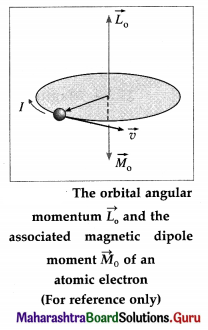L0 = mevr = $$\frac{nh}{2 \pi}$$ …………… (6)
Substituting for L0 in Eq. (4),
M0 = $$\frac{e n h}{4 \pi m_{\mathrm{e}}}$$
For n = 1, M0 = $$\frac{e n h}{4 \pi m_{\mathrm{e}}}$$
The quantity $$\frac{e n h}{4 \pi m_{\mathrm{e}}}$$ is a fundamental constant called the Bohr magneton,
µB ∙ µB = 9.274 × 10-24 J/T (or A∙m2) = 5.788 × 10-5 eV/T.
[ Notes : (1) Magnetic dipole moment is conventionally denoted by µ. (2) The magnetic moment of an atom is expressed in terms of Bohr magneton (vµB). (3) According to quantum mechanics, an atomic electron also has an intrinsic spin angular momentum and an associated spin magnetic moment of magnitude µ5. It is this spin magnetic moment that gives rise to magnetism in matter. (4) The total magnetic moment of the atom is the vector sum of its orbital magnetic moment and spin magnetic moment.]vi) What does the hysteresis loop represents?
A magnetic hysteresis loop is a closed curve obtained by plotting the magnetic flux density B of a ferromagnetic material against the corresponding magnetizing field H when the material is taken through a complete magnetizing cycle. The area enclosed by the loop represents the hysteresis loss per unit volume in taking the material through the magnetizing cycle.

vii) Explain one application of electromagnet.
Applications of an electromagnet:

1. Electromagnets are used in electric bells, loud speakers and circuit breakers.
2. Large electromagnets are used in junkyard cranes and industrial cranes to lift iron scraps.
3. Superconducting electromagnets are used in MRI and NMR machines, as well as in particle accelerators of cyclotron family.
4. Electromagnets are used in data storage devices such as computer hard disks and magnetic tapes.

Question 3.
When a plate of magnetic material of size 10 cm × 0.5 cm × 0.2 cm (length , breadth and thickness respectively) is located in magnetising field of 0.5 × 104 Am-1 then a magnetic moment of 0.5 A∙m2 is induced in it. Find out magnetic induction in plate.
Data : l = 10 cm, b = 0.5 cm, h = 0.2 cm,
H = 0.5 × 104 Am-1, M = 5 A∙m2
The volume of the plate,
V = 10 × 0.5 × 0.2 = 1 cm2 = 10-6 m2
B = μ0 (H + Mz) = μ0 (H + $$\frac{M}{V}$$)
The magnetic induction in the plate,
∴ B = 4π × 10-7 (0.5 × 104 + $$\frac{5}{10^{-6}}$$)
= 6.290 T

Question 4.
A rod of magnetic material of cross section 0.25 cm2 is located in 4000 Am-1 magnetising field. Magnetic flux passing through the rod is 25 × 10-6 Wb. Find out (a) relative permeability (b) magnetic susceptibility and (c) magnetisation of the rod.
Data: A = 0.25 cm2 = 25 × 10-6 m2,
H = 4000 A∙m-1, Φ = 25 × 10-6 Wb
Magnetic induction is
B = $$\frac{\phi}{A}=\frac{25 \times 10^{-6}}{25 \times 10^{-6}}$$ = 1 Wb/m2
(a) B = µ0µrK
∴ The relative permeability of the material,
µr = $$\frac{B}{\mu_{0} H}=\frac{1}{4 \times 3.142 \times 10^{-7} \times 4000}$$
= $$\frac{10000}{50.272}$$ = 198.91 = 199

(b) µr = 1 + χm
∴ The magnetic susceptibility of the material,
χm = µr – 1 = 199 – 1 = 198

(c) χm = $$\frac{M_{\mathrm{z}}}{H}$$
The magnetization of the rod,
Mz = χmH = 198 × 4000 = 7.92 × 105 A/mQuestion 5.
The work done for rotating a magnet with magnetic dipole momentm, through 90° from its magnetic meridian is n times the work done to rotate it through 60°. Find the value of n.
Data : θ0 = 0°, θ1 = 90°, θ2 = 60°, W1 = nW2
The work done by an external agent to rotate the magnet from θ0 to θ is
W = MB (cos θ0 – cos θ)
∴ W1 = MB(cos θ0 – cosθ1)
= MB (cos 0° – cos 90°)
= MB (1 – 0)
= MB

∴ W2 = MB (cos 0°- cos 60°)
= MB(1 – $$\frac{1}{2}$$)
= 0.5MB
∴ W1 = 2W2 = MB
Given W1 = nW2. Therefore n = 2.

Question 6.
An electron in an atom is revolving round the nucleus in a circular orbit of radius 5.3 × 10-11 m, with a speed of 2 × 106 ms-1 Find the resultant orbital magnetic moment and angular momentum of electron. (charge on electron e = 1.6 × 10-19 C, mass of electron me = 9.1 × 10-31 kg.)
Data: r = 5.3 × 10-11 m, v = 2 × 106 m/s,
e = 1.6 × 10-19 C, me = 9.1 × 10-31 kg
The orbital magnetic moment of the electron is
M0 = $$\frac{1}{2}$$ evr
= $$\frac{1}{2}$$ (1.6 × 10-19) (2 × 106) (5.3 × 10-11)
= 8.48 × 10-24 A∙m2
The angular momentum of the electron is
L0 = mevr
=(9.1 × 10-31) (2 × 106) (5.3 × 10-11)
= 96.46 × 10-36 = 9.646 × 10-35 kg∙m2/sQuestion 7.
A paramagnetic gas has 2.0 × 1026 atoms/m with atomic magnetic dipole moment of 1.5 × 10-23 A m2 each. The gas is at 27° C. (a) Find the maximum magnetization intensity of this sample. (b) If the gas in this problem is kept in a uniform magnetic field of 3 T, is it possible to achieve saturation magnetization? Why? (kB = 1.38 × 10-23 JK-1)[Answer: 3.0× 103 A m-1, No]
(Hint: Find the ratio of Thermal energy of atom of a gas ( 3/2 kBT) and maximum potential energy of the atom (mB) and draw your conclusion)
Data: $$\frac{N}{V}$$ = 2.0 × 1026 atoms/m3,
μ = 1.5 × 10-23 Am2, T = 27 + 273 = 300 K,
B = 3T, kB = 1.38 × 10-23 J/K, 1 eV = 1.6 × 10-19 J
(a) The maximum magnetization of the material,
Mz = $$\frac{N}{V}$$μ =(2.0 × 1026) (1.5 ×10-23)
= 3 × 103 A/m

(b) The maximum orientation energy per atom is
Um = -μB cos 180° = μB
= (1.5 × 10-23) (3) = $$\frac{4.5 \times 10^{-23}}{1.6 \times 10^{-19}}$$
= 2.8 × 10-4 eV

The average thermal energy of each atom,
E = $$\frac{3}{2}$$ kBT
where kB is the Botzmann constant.
∴ E = 1.5(1.38 × 10-23)(300)
= 6.21 × 10-21 J = $$\frac{6.21 \times 10^{-21}}{1.6 \times 10^{-19}}$$
= 3.9 × 10-2 eV
Since the thermal energy of randomization is about two orders of magnitude greater than the magnetic potential energy of orientation, saturation magnetization will not be achieved at 300 K.

Question 8.
A magnetic needle placed in uniform magnetic field has magnetic moment of 2 × 10-2 A m2, and moment of inertia of 7.2 × 10-7 kg m2. It performs 10 complete oscillations in 6 s. What is the magnitude of the magnetic field ?
Data: M = 2 × 10-2 A∙m2, I = 7.2 × 10-7 kg∙m2,
T = $$\frac{6}{10}$$ = 0.6 S
T = 2π$$\sqrt{\frac{I}{M B}}$$
The magnitude of the magnetic field is
B = $$\frac{4 \pi^{2} I}{M T^{2}}$$
= $$\frac{(4)(3.14)^{2}\left(7.2 \times 10^{-7}\right)}{\left(2 \times 10^{-2}\right)(0.6)^{2}}$$
= 3.943 × 10-3 T = 3.943 mT

Question 9.
A short bar magnet is placed in an external magnetic field of 700 guass. When its axis makes an angle of 30° with the external magnetic field, it experiences a torque of 0.014 Nm. Find the magnetic moment of the magnet, and the work done in moving it from its most stable to most unstable position.
Data : B = 700 gauss = 0.07 tesla, θ = 30°,
τ = 0.014 N∙m
τ = MB sin θ
The magnetic moment of the magnet is
M = $$\frac{\tau}{B \sin \theta}=\frac{(0.014)}{(0.07)\left(\sin 30^{\circ}\right)}$$ = 0.4 A∙m2
The most stable state of the bar magnet is for θ = 0°.
It is in the most unstable state when θ = 180°. Thus, the work done in moving the bar magnet from 0° to 180° is
W = MB(cos θ0 – cos θ)
= MB (cos 0° – cos 180°)
= MB [1 – (-1)]
= 2 MB = (2) (0.4) (0.07)
= 0.056 J
This the required work done.Question 10.
A magnetic needle is suspended freely so that it can rotate freely in the magnetic meridian. In order to keep it in the horizontal position, a weight of 0.1 g is kept on one end of the needle. If the pole strength of this needle is 20 Am , find the value of the vertical component of the earth’s magnetic field. (g = 9.8 m s-2)
Data: M = 0.2g = 2 × 10-4kg, qm = 20 A∙m, g =9.8 m/s2

Without the added weight at one end, the needle will dip in the direction of the resultant magnetic field inclined with the horizontal. The torque due to the added weight about the vertical axis through the centre balances the torque of the couple due to the vertical component of the Earth’s magnetic field.
∴ (Mg)$$\left(\frac{L}{2}\right)$$ = (qm Bv) L
The vertical component of the Earth’s magnetic field,
Bv = $$\frac{M g}{2 q_{\mathrm{m}}}=\frac{\left(2 \times 10^{-4}\right)(9.8)}{2(20)}$$ = 4.9 × 10-5 T

Question 11.
The susceptibility of a paramagnetic material is χ at 27° C. At what temperature its susceptibility be $$\frac{\chi}{3}$$ ?
Data: χm1 = χ, T1 = 27°C = 300 K, χm2 = $$\frac{\chi}{3}$$
By Curie’s law,
Mz = C$$\frac{B_{0}}{T}$$
Since Mz = χmH = B0 = μ0H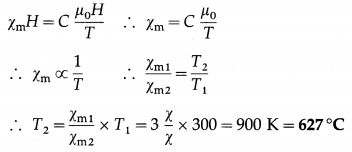This gives the required temperature.

12th Physics Digest Chapter 11 Magnetic Materials Intext Questions and Answers

Activity (Textbook Page No. 251)

Question 1.
You have already studied in earlier classes that a short bar magnet suspended freely always aligns in North South direction. Now if you try to forcefully move and bring it in the direction along East West and leave it free, you will observe that the magnet starts turning about the axis of suspension. Do you know from where does the torque which is necessary for rotational motion come from? (as studied in rotational dynamics a torque is necessary for rotational motion).
Suspend a short bar magnet such that it can rotate freely in a horizontal plane. Let it come to rest along the magnetic meridian. Rotate the magnet through some angle and release it. You will see that the magnet turns about the vertical axis in trying to return back to its equilibrium position along the magnetic meridian. Where does the torque for the rotational motion come from?

Take another bar magnet and bring it near the suspended magnet resting in the magnetic meridian. Observe the interaction between the like and unlike poles of the two magnets facing each other. Does the suspended magnet rotate continuously or rotate through certain angle and remain stable? Note down your observations and conclusions.Do you know (Textbook Page No. 255)

Effective magneton numbers for iron group ions (No. of Bohr magnetons)

 Ion Electron configuration Magnetic moment (in terms of /iB) Fe3 + [Ar] 3s23p63d5 5.9 Fe2 + [Ar] 3s23p63d6 5.4 Co2 + [Ar] 3s23p63d7 4.8 n2+ [Ar] 3s23p63d8 3.2

(Courtsey: Introduction to solid state physics by Charles Kittel, pg. 306 )
These magnetic moments are calculated from the experimental value of magnetic susceptibility. In several ions the magnetic moment is due to both orbital and spin angular momenta.
In terms of Bohr magneton (µB), the effective magnetic moments of some iron group ions are as follows. In several cases, the magnetic moment is due to both orbital and spin angular momenta.

 Ion Configuration Effective magnetic moment in terms of Bohr magneton (B.M) (Expreimental values) Fe3 + 3d5 5.9 Fe2 + 3d6 5.4 Co2 + 3d7 4.8 n2+ 3d8 3.2

Remember this (Textbook Page No. 256)

Question 1.
Permeability and Permittivity:
Magnetic Permeability is a term analogous to permittivity in electrostatics. It basically tells us about the number of magnetic lines of force that are passing through a given substance when it is kept in an external magnetic field. The number is the indicator of the behaviour of the material in magnetic field. For superconductors χ = – 1. If you substitute in the Eq. (11.18), it is observed that permeability of material µ = 0. This means no magnetic lines will pass through the superconductor.

Magnetic Susceptibility (χ) is the indicator of measure of the response of a given material to the external applied magnetic field. In other words it indicates as to how much magnetization will be produced in a given substance when kept in an external magnetic field. Again it is analogous to electrical susceptibility. This means when the substance is kept in a magnetic field, the atomic dipole moments either align or oppose the external magnetic field. If the atomic dipole moments of the substance are opposing the field, χ is observed to be negative, and if the atomic dipole moments align themselves in the direction of field, χ is observed to be positive. The number of atomic dipole moments of getting aligned in the direction of the applied magnetic field is proportional to χ. It is large for soft iron (χ >1000).
Magnetic permeability is analogous to electric permittivity, both indicating the extent to which a material permits a field to pass through or permeate into the material. For a superconductor, χ = -1 which makes µ = 0, so that a superconductor does not allow magnetic field lines to pass through it.

Magnetic susceptibility (χ). analogous to electrical susceptibility, is a measure of the response of a given material to an applied magnetic field. That is, it indicates the extent of the magnetization produced in the material when it is placed in an external magnetic field. χ is positive when the atomic dipole moments align themselves in the direction of the applied field; χ is negative when the atomic dipole moments align antiparallel to the field. χ is large for soft iron (χ > 1000).Use your brain power (Textbook Page No. 259)

Question 1.
Classify the following atoms as diamagnetic or paramagnetic.
H, O, Zn, Fe, F, Ar, He
(Hint : Write down their electronic configurations)
Is it true that all substances with even number of electrons are diamagnetic?

 Atoms Electronic configuration No. of Electrons Diamagnetic/Paramagnetic H 1s1„ 1 Diamagnetic 0 1s22s22p4 8 Paramagnetic Zn 1s22s22p63s23p63d104s2 30 Diamagnetic Fe 1s22s22p63s23p64s23d6 26 Neither diamagnetic nor paramagnetic (ferromagnetic) F 1s22s22p5 9 Paramagnetic Ar 1s22s22p63s23p6 18 Diamagnetic He Is2 2 Diamagnetic

It can be seen that all substances with an even number of electrons are not necessarily diamagnetic.

Do you know (Textbook Page No. 260)

Question 1.
Exchange Interaction: This exchange interaction in stronger than usual dipole-dipole interaction by an order of magnitude. Due to this exchange interaction, all the atomic dipole moments in a domain get aligned with each other. Find out more about the origin of exchange interaction.
Exchange Interaction :
Quantum mechanical exchange interaction be-tween two neighbouring spin magnetic moments in a ferromagnetic material arises as a consequence of the overlap between the magnetic orbitals of two adjacent atoms. The exchange interaction in particular for 3d metals is stronger than the dipole-dipole interaction by an order of magnitude. Due to this, all the atomic dipole moments in a domain get aligned with each other and each domain is spontaneously magnetized to saturation. (Quantum mechanics and exchange interaction are beyond the scope of the syllabus.)

Use your brain power (Textbook Page No. 262)

Question 1.
What does the area inside the curve B – H (hysteresis curve) indicate?
A magnetic hysteresis loop is a closed curve obtained by plotting the magnetic flux density B of a ferromagnetic material against the corresponding magnetizing field H when the material is taken through a complete magnetizing cycle. The area enclosed by the loop represents the hysteresis loss per unit volume in taking the material through the magnetizing cycle.

Do you know (Textbook Page No. 262)

Question 1.
What is soft magnetic material?
Soft ferromagntic materials can be easily magnetized and demagnetized.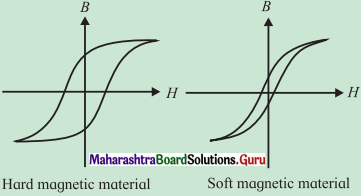Hysteresis loop for hard and soft ferramagnetic materials.